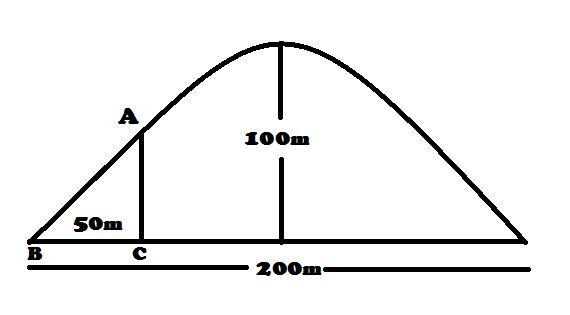# Aditya's challenges in Mechanics 3In the competition of Tour de France, the cyclists have to go over a parabolic hill. The leading cyclist is riding at a constant speed $v=10\sqrt{5} \text{ ms}^{-1}$. The details of the hill are depicted in the above figure. Find the net force applied by the hill at point $A$

Details and Assumptions

• The total mass of of the bicycle and the cyclist is equal $100\sqrt{\frac{2}{5}} \text {kg}$.

• $g=10 \text{ ms}^{-2}$.

• The shape of the hill is similar to that of a parabola.

Try more here.

×